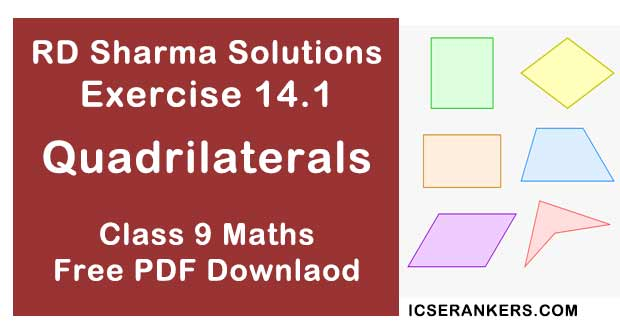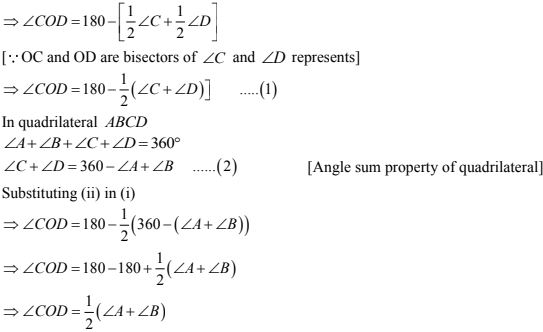# Chapter 14 Quadrilaterals RD Sharma Solutions Exercise 14.1 Class 9 MathsChapter Name RD Sharma Chapter 14 Quadrilaterals Exercise 14.1 Book Name RD Sharma Mathematics for Class 10 Other Exercises Exercise 14.2Exercise 14.3Exercise 14.4 Related Study NCERT Solutions for Class 10 Maths

### Exercise 14.1 Solutions

1. Three angles of a quadrilateral are respectively equal to 110° , 50° and 40° . Find its fourth angles.

Solution

Given,
Three angles are 110°, 50° and 40°
Let fourth angle be x
We have,
Sum of all angles of a quadrilaterals = 360°
110° + 50° + 40° +x° = 360°
⇒ x = 360° - 200°
⇒ x = 160°
Required fourth angle  = 160°

2. In a quadrilateral ABCD, the angles A, B, C and D are in the ratio 1 : 2 : 4 : 5. Find the measure of each angles of the quadrilateral.

Solution

Let the angles of the quadrilateral be
A = x, B = 2x, C = 4x and D = 5x then,
A + B + C + D = 360°
⇒ x + 2x + 4x + 5x = 360°
⇒ 12x = 360°
⇒ x = 360°/12
⇒ x = 30°
∴A = x = 30°
B = 2x = 60°
C = 4x = 30° (4)= 120°
D = 5x = 5(30°) = 150°

3. In a quadrilateral ABCD, CO and DO are the bisectors of ∠C and ∠D respectively. Prove that ∠COD = 1/2 (∠A + ∠B).

Solution

In ΔDOC
∠1 + ∠COD + ∠2 = 180°  [Angle sum property of a triangle]
⇒ ∠COD = 180 - ∠1 - ∠2
⇒ ∠COD = 180 - ∠1 + ∠24. The angles of quadrilateral are in the ratio 3 : 5 : 13. Find all the angles of the quadrilateral.

Solution

Let the common ratio between the angle is 'x' so the angles will be 3x, 5x, 9x and 13x respectively
Since the sum of all interior angles of a quadrilateral is 360°
∴ 3x + 5x + 9x + 13x = 360°
⇒ 30x  = 360°
⇒ x = 12°
Hence, the angles are
3x = 3×12 = 36°
5x = 5× 12 = 60°
9x = 9 × 12= 108°
13x = 13 × 12 = 156°